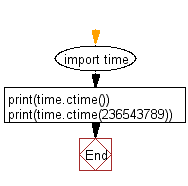﻿ Python: Convert a given time in seconds since the epoch to a string representing local time - w3resource

# Python: Convert a given time in seconds since the epoch to a string representing local time

## Python Datetime: Exercise-59 with Solution

Write a Python program to convert a given time in seconds since the epoch to a string representing local time.

Sample Solution:

Python Code:

``````import time
print(time.ctime())
print(time.ctime(236543789))
```
```

Sample Output:

```Tue Apr 13 11:51:51 2021
Thu Jun 30 18:36:29 1977
```

Flowchart:Python Code Editor: